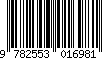Applied Reactor Physics (2nd Ed.)Cursus Series

Auteur : HÉBERT Alain

Langue : AnglaisThèmes d'Applied Reactor Physics :

Date de parution :
Ouvrage 396 p. · 17x24.5 cm · Broché ·
ISBN : 9782553016981Presses internationales Polytechnique

• Biographie

Reactor physics is the discipline devoted to the study of interactions between neutrons and matter in a nuclear reactor. In Applied Reactor Physics, reactor physics is approached from the fundamental level. Legacy numerical techniques are introduced with sufficient details to permit their implementati on in Matlab. More advanced and/or proprietary techniques may be available in a production environment, but these can be obtained as evolutions of the fundamental approaches presented in the book.

A characteristic of Applied Reactor Physics is to emphasize the
algorithmic nature of numerical solution techniques used in reactor
physics. Many numerical soluti on approaches described in the book are
accompanied by Matlab scripts and readers are encouraged to write short
Matlab scripts of their own in order to solve the Endof-Chapter exercises.
Chapter 1 - Introduction

Chapter 2 - Cross sections and nuclear data
Solid angles and spherical harmonics
Dealing with distributions
Dynamics of a scattering reaction
Definition of a cross section
Formation of a compound nucleus
Thermal agitation of nuclides and binding effects
Expansion of the differential cross sections
Calculation of the probability tables
Production of an isotopic cross-section library
Exercises

Chapter 3 - The transport equation
The particle flux
Derivation of the transport equation
Source density in reactor physics
The transport correction
Multigroup discretization
The first-order streaming operator
The spherical harmonicsmethod
The collision probability method
The discrete ordinatesmethod
The method of characteristics
The multigroupMonte Carlomethod
Exercises

Chapter 4 - Elements of lattice calculation
A historical overview
Neutron slowing-down and resonance self-shielding
The neutron leakage model
The SPH equivalence
technique
Isotopic depletion
Creation of the reactor database
A presentation of DRAGON
Exercises

Chapter 5 - Full-core calculations

The steady-state diffusion equation
Discretization of the neutron diffusion equation
Generalized perturbation theory
Space-time kinetics
Exercises